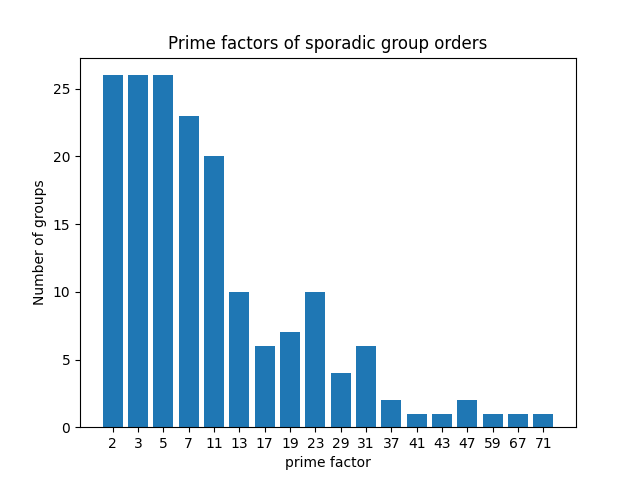# Factors of orders of sporadic groups

I was curious about the orders of the sporadic groups, specifically their prime factors, so I made a bar graph to show how often each factor appears.

To back up a little bit, simple groups are to groups roughly what prime numbers are to integers. Finite simple groups have been fully classified, and they fall into several infinite families of groups, except for 26 misfits, the so-called sporadic groups. The largest of these is nicknamed The Monster and has surprising connections to various areas of math.One thing that jumps out  is that the order of every sporadic group is divisible by 2, 3, and 5. (In fact they’re all divisible by 120, i.e. by 2³, 3, and 5.) Another is that all the primes between 2 and 71 appear somewhere, except 53 and 61 are missing.

I don’t know whether there’s any significance to these patterns. Maybe they’re a simple consequence of things that people who work in this area know. I’m just noodling around here.

The Python code that produced the plot is given below.

## Related posts

```from sympy import primefactors
from collections import Counter
import matplotlib.pyplot as plt

orders = [
95040,
175560,
443520,
604800,
10200960,
17971200,
44352000,
50232960,
244823040,
898128000,
4030387200,
145926144000,
448345497600,
460815505920,
95766656000,
42305421312000,
64561751654400,
273030912000000,
51765179004000000,
90745943887872000,
4089470473293004800,
4157776806543360000,
86775571046077562880,
1255205709190661721292800,
4154781481226426191177580544000000,
808017424794512875886459904961710757005754368000000000
]

c = Counter()
for o in orders:
for f in primefactors(o):
c[f] += 1

factors = sorted(c.keys())
frequencies = [c[k] for k in factors]

fig, ax = plt.subplots()
ax.bar([str(f) for f in factors], frequencies)
ax.set_xlabel("prime factor")
ax.set_ylabel("Number of groups")
ax.set_title("Prime factors of sporadic group orders")
```